# NCERT Solutions For Class 6 Maths Practical Geometry Exercise 14.4

NCERT Solutions For Class 6 Maths Practical Geometry Exercise 14.4

### NCERT Solutions For Class 6 Maths Practical Geometry Exercise 14.4

NCERT Solutions For Class 6 Maths Chapter 14 Practical Geometry Ex 14.4

Exercise 14.4

Ex 14.4 Class 6 Maths Question 1.Step III : Take P and Q as centres and radius greater than PM, draw two arcs such that they intersect each other at C.
Step IV : Join M and C.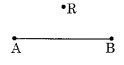Step IV : Hold the ruler fix and slide the set square along the ruler till it touches the point R.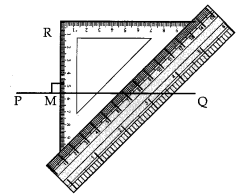Ex 14.4 Class 6 Maths Question 3.Solution:
Step I: Draw a line l and take a point X on it.
Step II : Draw an arc with centre X and of suitable radius to intersect the line l at two points P and Q.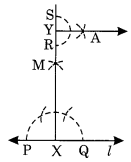Step III : With P and Q as centres and a radius greater than P draw two arcs to intersect each other at M.
Step IV : Join XM and produce to Y.
Step V : With Y as centre and a suitable radius, draw an arc to intersect XY at two points R and S.
Step VI: With R and S as centres and a radius greater than YR, draw two arcs to intersect each other at A.
Step VII: Join Y and A. Thus YA ⊥ XY.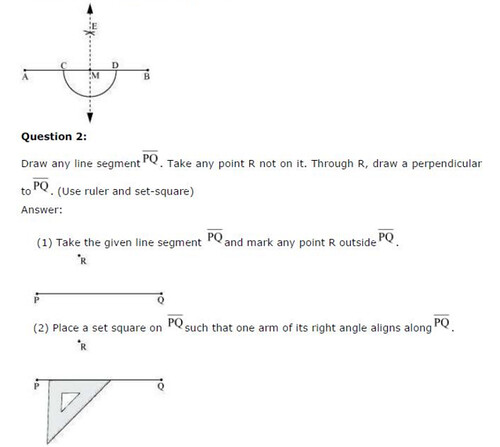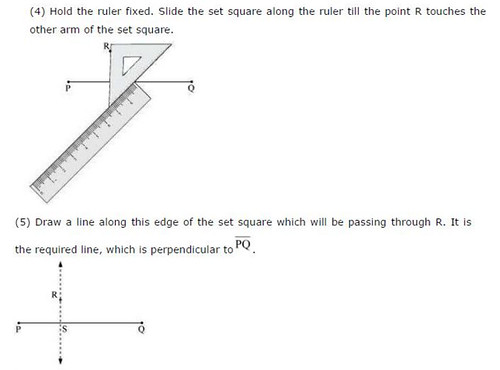## SabDekho

The Complete Educational Website